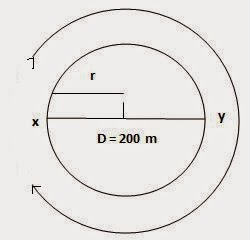#### NCERT Exercise

An athlete completes one round of a circular track of diameter 200 m in 40 s. What will be the distance covered and the displacement at the end of 2 minutes 20 s?
Diameter of circular track (D) = 200 m
Radius of circular track (r) = 200 / 2=100 m
Time taken by the athlete for one round (t) = 40 s
Distance covered by athlete in one round (s) = 2π r
= 2 � ( 22 / 7 ) � 100
Speed of the athlete (v) = Distance / Time
= (2 � 2200) / (7 � 40)
= 4400 / 7 � 40
Therefore, Distance covered in 140 s = Speed (s) � Time(t)
= 4400 / (7 � 40) � (2 � 60 + 20)
= 4400 / (7 � 40) � 140
= 4400 � 140 /7 � 40
= 2200 m

Number of round in 40 s =1 round
Number of round in 140 s =140/40
=3 1/2
After taking start from position X,the athlete will be at postion Y after 3 1/2 rounds as shown in figureHence, Displacement of the athlete with respect to initial position at x= xy
= Diameter of circular track
= 200 m

###### Latest Post
How do Organisms Reproduce?
People as Resource
Why Do We Fall ill
Areas of Parallelograms and Triangles
Pair of Linear Equations in Two Variables